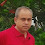Latest Articles

# Ultimate and Twice the Penultimate Vedic Sutra -Sopantyadvayamantyam

Info Post
The Ultimate and Twice the Penultimate also known as 'Sopantyadvayamantyam" is the Thirteenth sutra in the Vedic Maths and it helps in solving problems immediately.Let us take any multiplication of 12 .

Example: 132 * 12 =?

Step 1 :
In this example, we are going to make a zero sandwich for the number 132 as follows
o 132 o

Step 2:
The above answer is our starter, from where we keep adding the last digit  and twice the second last digit .That means, we proceed for  o132o as follows

Last digit is "o" + Twice the second last digit " 2 "
= 0 + (2*2)
= 4      ------ (1)

Step 3:
Now we add the last digit 2 plus twice the second last digit again for the o132o

Last digit "2" + Twice the second last digit "3"
= 2 + ( 2 * 3)
= 8     ------- (2)

Step 4 :
Repeating the same steps as explained above,

Last digit "3" +Twice the second last digit "1"
= 3 + ( 2 * 1)
= 5   ...........(3)

Step 5:
Again,repeating the same steps

Last digit "1" + Twice the second last digit "0"
= 1   .........(4)

Step 6 :
Accumulating the derived answer in order as we move from left to right we , get

132 * 12 = (4)(3)(2)(1)

Therefore , 132  * 12 =1584

You can try it similarly for any other multiplication problems concerning 12 :).Visit this blog again , more on Vedic Maths to be blogged with more interesting sutra's .

Share:

1.Interesting

2.Do you know that there is nothing vedic in vedic math.There is no such sutras in veda.Do you know that fractions came into reality after 12th century and vedas are there from much before that.These are only tricks.Useful yes but vedic!no.

3.Anonymous15 May, 2012

dont you talk like the foolish englishmen ,
the sutras are condensed in the atharva veda and even if you dont belive ask a scholar of vedas

4.@anonymous - Vedic Maths is vast like ocean and there are many forms of Vedic literature like Rig Veda, Yajur Veda,Sama Veda and Atharva Veda.

What I have written here so far is the Maths that is based on the 16 sutras under Vedic system developed by Bharati Krishna Tirthaji.

Btw, I have given you the straight methods of calculation using the sutras and not any theory behind this .

5.this is not been applied to 187*12 so please give a method to explain it briefly

6.@sanjeev jain

This applies to 187 * 12 as well .Since the answers are two digits numbers , we have to carry over as explained (in my other examples in this blog ) below ,

187 * 12

[ Doing as the same as the above ]

last digit + 2( second last digit )

0 187 0

0 + 14 = 14 -->(1)

7 + 16 = 23 -->(2)

8 + 2 = 10 -->(3)

1 + 0 = 1 -->(4)

Accumulating the results above from (4)to (1),

= 1 |10 | 23 | 14

Here, carry over the second digit to the next (to left) keeping the first digit as such ,

= 2244

Therefore , 187 * 12 = 2244

:)

Thank You for visiting Momscribe.com !